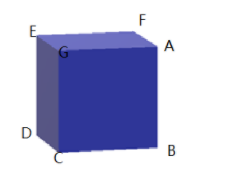QuestionAnswers

# The number of corners in a cube areA) 4B) 6C) 8D) 12

Hint: A cube is a solid shape with all surfaces as square which are identical. An edge is there when two faces meet. And the meeting point of two or more edges is known as the corner or vertex of the given shape. Determine the number of such corners present in the cube.

Complete step by step solution:
A cube is a 3- dimensional figure. All the sides of a square are equal. Its base is a square.The square surfaces are also known as faces. A cube has 6 faces.
For example, $\text{EGCDE}$ is surface and is square in shape.
Also, the edge is a line segment where two faces meet.
Here in the above figure, $\text{AB, BC, CG, EG}$ etc. are all edges in the above figure.
As, we can see, in a cube, all the faces are identical.
In a cube, there are 12 edges and the edges are of equal size.
And a corner is the joining point or meeting point of two or more edges. Corners are also known as vertices.
In a cube, three edges meet to form a point known as corner or vertex.
For example, edges $\text{CG, EG and AG}$ join to form vertex $\text{G}$ in the above figure.
There are 8 corners in a cube.
Thus, the number of corners in a cube are 8

Hence, option C is correct.

Note: A cube is a 3- dimensional figure with square base. All the edges of a square are equal. A corner is the intersection point of two or more edges. A corner is known as vertex. Thus, a cube has 6 faces, 12 edges and 8 vertices.